# Rate of Change Chapter Exam

Exam Instructions:

Choose your answers to the questions and click 'Next' to see the next set of questions. You can skip questions if you would like and come back to them later with the yellow "Go To First Skipped Question" button. When you have completed the practice exam, a green submit button will appear. Click it to see your results. Good luck!

### Page 1

#### Question 1 1. How many zeroes does the derivative of this graph have?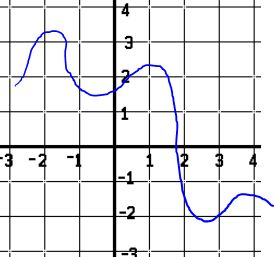#### Question 4 4. According to the mean value theorem, at how many points will the instantaneous rate of change equal the average rate of change between x = 1 and x = 3 on the following graph?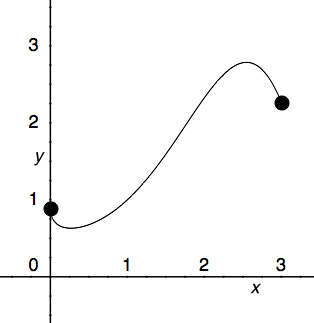#### Question 5 5. Which is greater, the derivative at a or the derivative at b?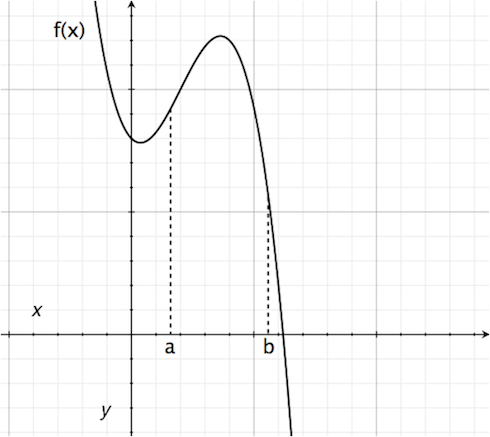### Page 2

#### Question 7 7. Where t= time, what is the derivative of f(t)?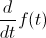#### Question 9 9. Where is f(x) differentiable?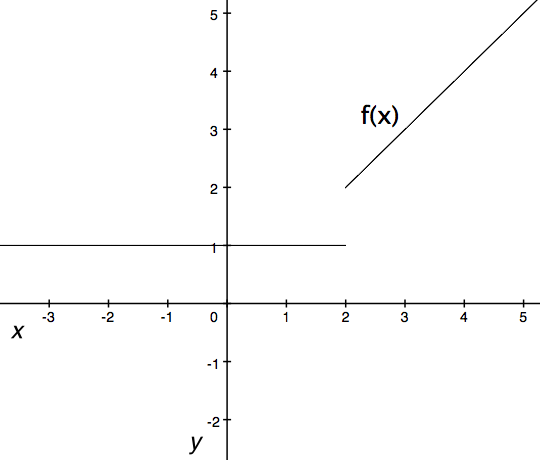#### Question 10 10. What is the rate of change at the point B in the graph below (for y in relation to x)?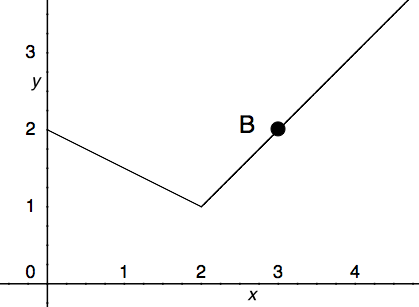### Page 3

#### Question 11 11. Where is f(x) differentiable?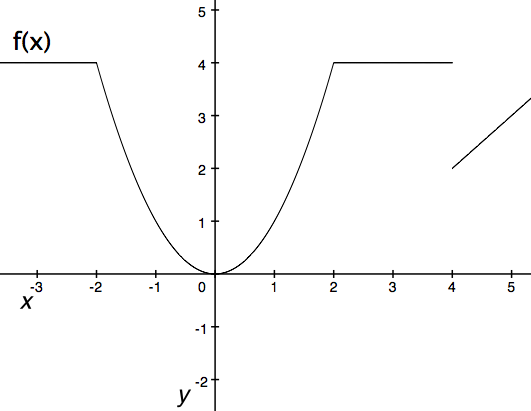#### Question 12 12. Consider the function f(x) given below. According to Rolle's theorem, for how many values of x is the instantaneous rate of change equal to 0?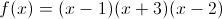#### Question 14 14. What is the rate of change at the point B in the graph below (for y in relation to x)? (In other words, what is the slope of the line from x = 2 to x = 3?)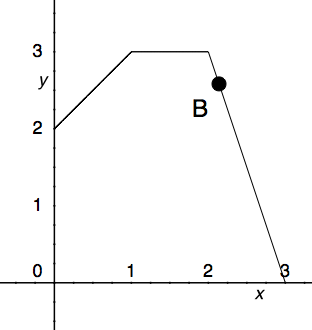### Page 4

#### Question 16 16. Does Rolle's theorem apply to this function?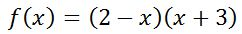#### Question 17 17. What is the rate of change at point A of the graph below.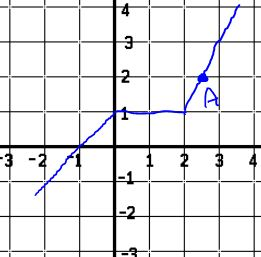#### Question 19 19. Is this function differentiable over the region beginning at x=-2 and ending at x=2?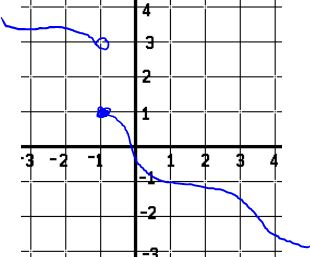#### Question 20 20. At how many points on the graph below is the derivative of f(x)=0?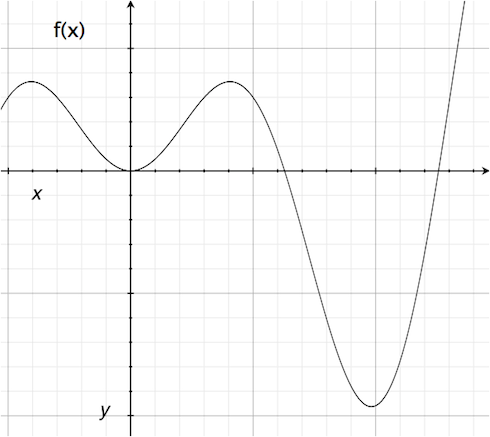### Page 5

#### Question 24 24. Consider the graph below. Between x=C and x=-C, for any value of C, will there be a point where the instantaneous rate of change is 0?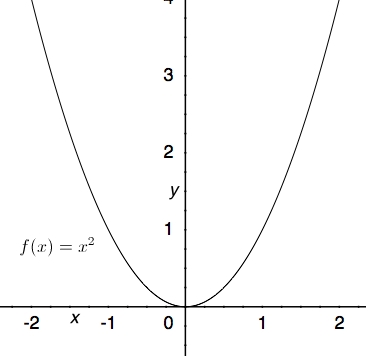#### Question 25 25. What is the rate of change at the point A in the graph below (for y in relation to x)?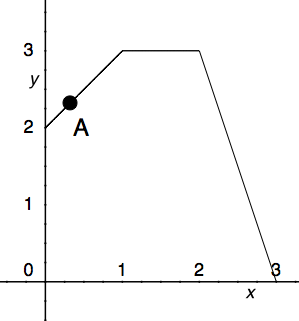### Page 6

#### Question 26 26. Which point on the following graph satisfies the mean value theorem between x = 0 and x = 3?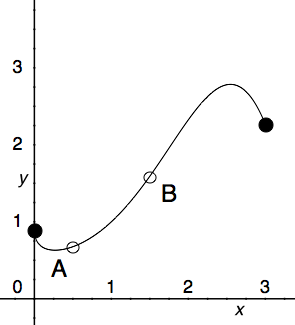#### Question 28 28. What is the average rate of change for the interval with endpoints A and B?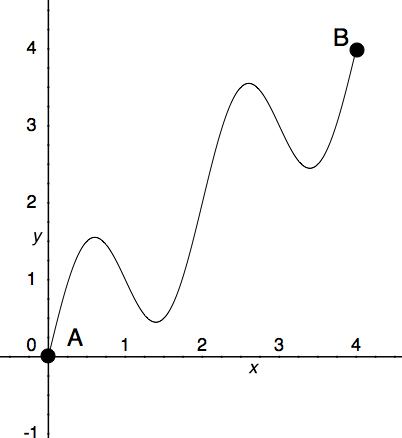#### Question 30 30. Consider the function f(x) given below. According to Rolle's theorem, between x=0 and x=pi, for how many values of x is the instantaneous rate of change equal to 0?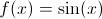#### Rate of Change Chapter Exam Instructions

Choose your answers to the questions and click 'Next' to see the next set of questions. You can skip questions if you would like and come back to them later with the yellow "Go To First Skipped Question" button. When you have completed the practice exam, a green submit button will appear. Click it to see your results. Good luck!

Support